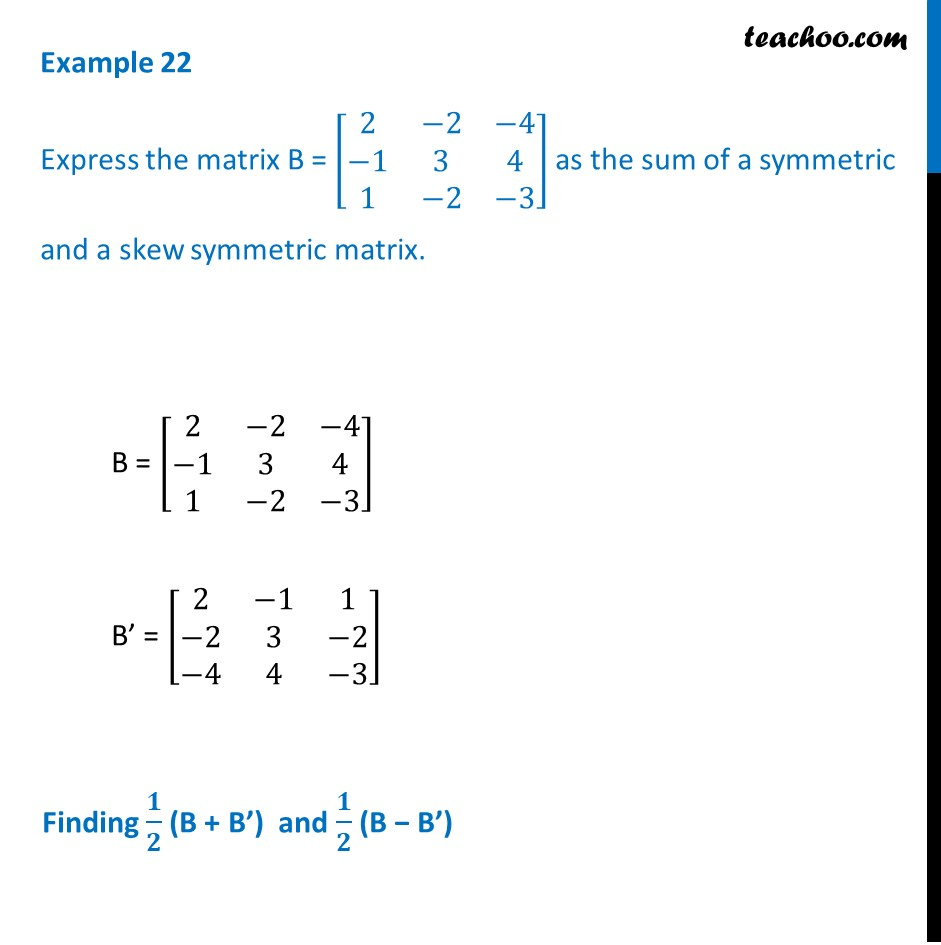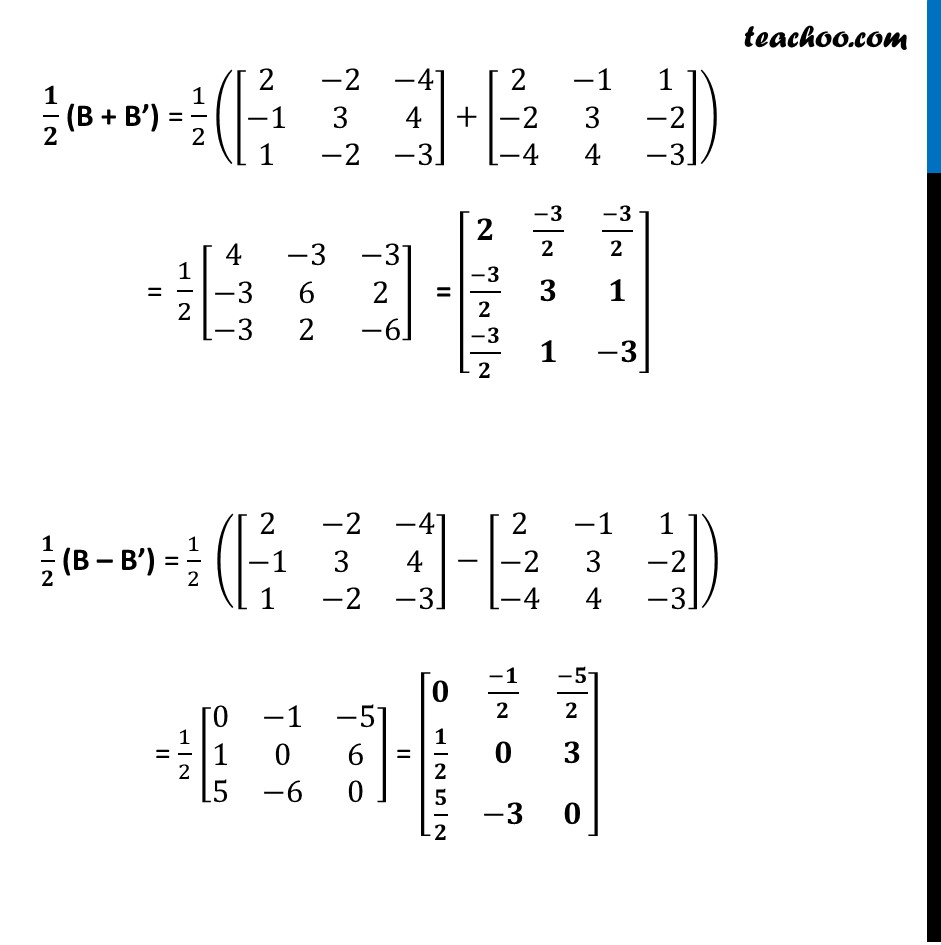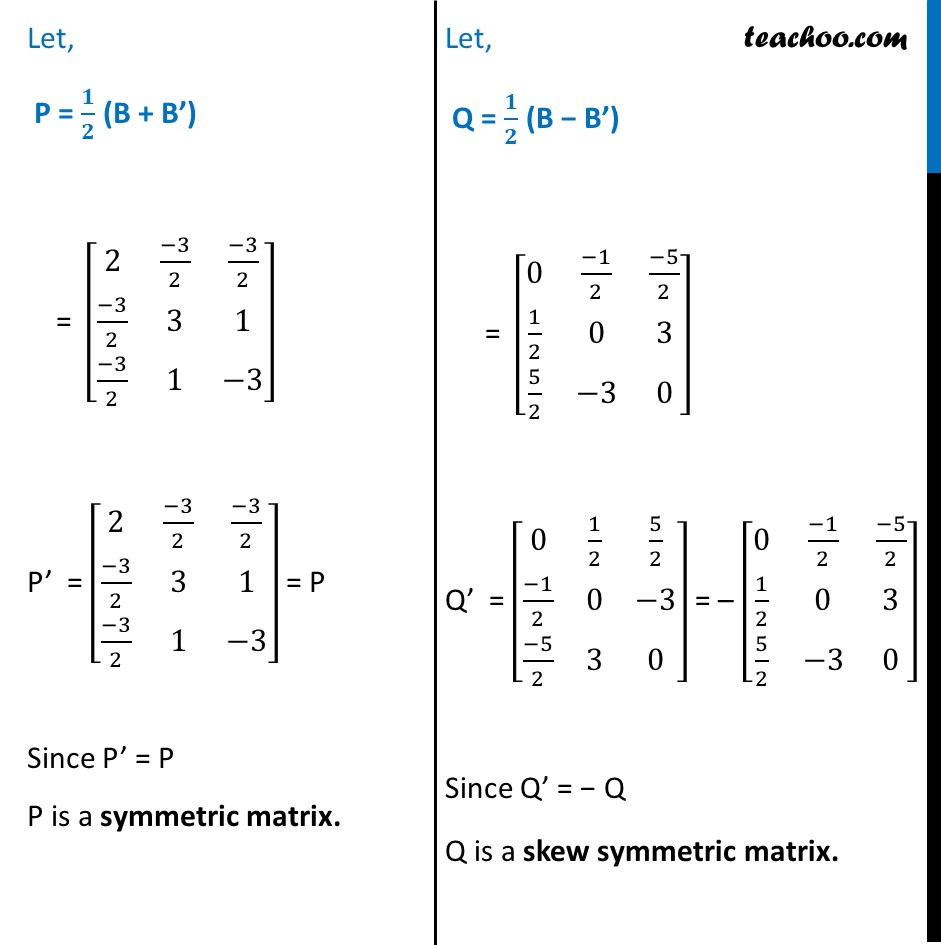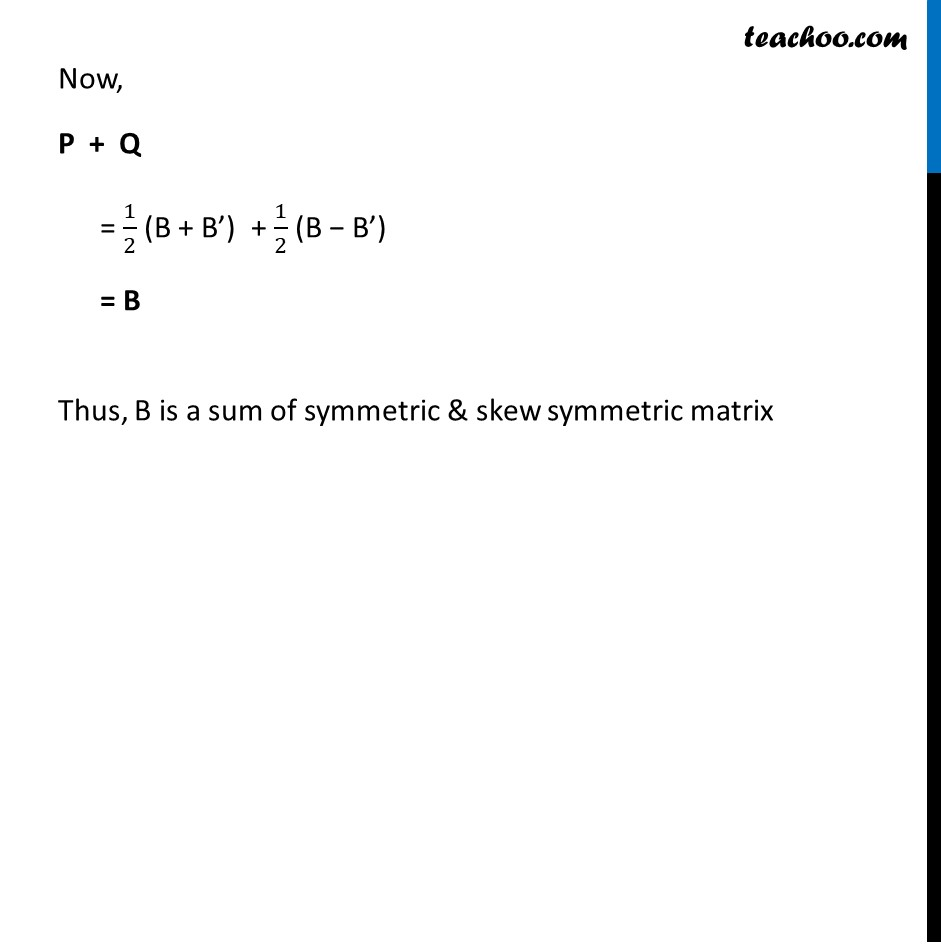Symmetric and skew symmetric matrices

Chapter 3 Class 12 Matrices
Concept wiseLearn in your speed, with individual attention - Teachoo Maths 1-on-1 Class

### Transcript

Example 22 Express the matrix B = [■8(2&−2&−4@−1&3&4@1&−2&−3)] as the sum of a symmetric and a skew symmetric matrix. B = [■8(2&−2&−4@−1&3&4@1&−2&−3)] B’ = [■8(2&−1&1@−2&3&−2@−4&4&−3)] Finding 𝟏/𝟐 (B + B’) and 𝟏/𝟐 (B − B’) 𝟏/𝟐 (B + B’) = 1/2 ([■8(2&−2&−4@−1&3&4@1&−2&−3)]+[■8(2&−1&1@−2&3&−2@−4&4&−3)]) = 1/2 [■8(4&−3&−3@−3&6&2@−3&2&−6)] = [■8(𝟐&(−𝟑)/𝟐&(−𝟑)/𝟐@(−𝟑)/𝟐&𝟑&𝟏@(−𝟑)/𝟐&𝟏&−𝟑)] 𝟏/𝟐 (B – B’) = 1/2 ([■8(2&−2&−4@−1&3&4@1&−2&−3)]−[■8(2&−1&1@−2&3&−2@−4&4&−3)]) = 1/2 [■8(0&−1&−5@1&0&6@5&−6&0)] = [■8(𝟎&(−𝟏)/𝟐&(−𝟓)/𝟐@𝟏/𝟐&𝟎&𝟑@𝟓/𝟐&−𝟑&𝟎)] Let, P = 𝟏/𝟐 (B + B’) = [■8(2&(−3)/2&(−3)/2@(−3)/2&3&1@(−3)/2&1&−3)] P’ = [■8(2&(−3)/2&(−3)/2@(−3)/2&3&1@(−3)/2&1&−3)] = P Since P’ = P P is a symmetric matrix. Let, Q = 𝟏/𝟐 (B − B’) = [■8(0&(−1)/2&(−5)/2@1/2&0&3@5/2&−3&0)] Q’ = [■8(0&1/2&5/2@(−1)/2&0&−3@(−5)/2&3&0)] = – [■8(0&(−1)/2&(−5)/2@1/2&0&3@5/2&−3&0)] Since Q’ = − Q Q is a skew symmetric matrix. Now, P + Q = 1/2 (B + B’) + 1/2 (B − B’) = B Thus, B is a sum of symmetric & skew symmetric matrix